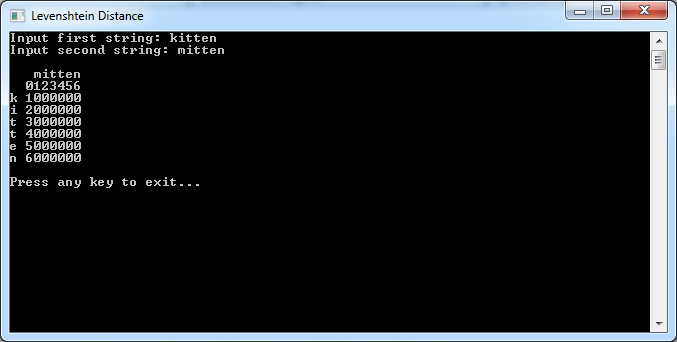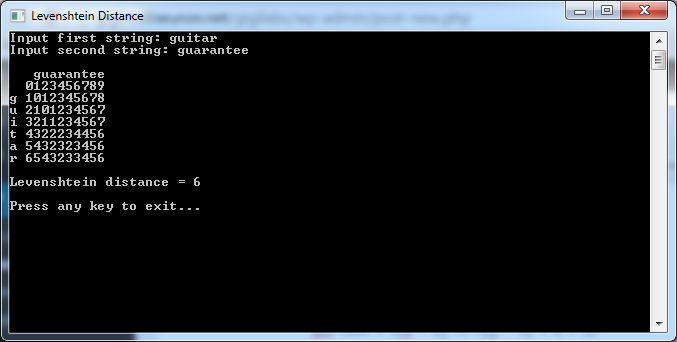# Calculating String Similarity with Levenshtein Distance

Levenshtein distance or edit distance is a metric used to measure the similarity between two strings. It works by calculating the number of edits needed to go from the first string to the second. For example:

• To go from kitten to mitten, we need to replace the first letter. That’s just one edit.
• To go from barrel to bard, we need to remove the last two letters, and replace an ‘r’ with a ‘d’. That’s three edits.
• To go from bled to bleeding, we need to insert an ‘e’ in the middle and “ing” at the end. That’s four edits.
• Transposition of characters also a valid operation.

To perform this calculation in code, there’s a simple algorithm. It starts off by initialising a matrix and setting initial values for the top row and left column. Let’s see how this looks in code.

We’ll start off with a simple prompt for the two strings to be compared:

```            // input

Console.Write("Input first string: ");
Console.Write("Input second string: ");
```

Next, we create our matrix and set initial values for the top row and left column:

```            // init

var matrix = new int[s.Length + 1, t.Length + 1];

for (int i = 0; i < s.Length + 1; i++)
matrix[i, 0] = i;

for (int j = 0; j < t.Length + 1; j++)
matrix[0, j] = j;
```

Rather than explaining what this does in words, it’s better if we just draw the matrix in the console window so you can see for yourself. To do that, we’ll need a helper function. This might look like a lot of code, but it’s basically just drawing the matrix with one string at the top and the other at the side:

```        static void DrawMatrix(string s, string t, int[,] matrix)
{
Console.WriteLine();
Console.Write("   ");

for (int i = 0; i < t.Length; i++)
Console.Write("{0}", t[i]);
Console.WriteLine();

for (int i = 0; i < s.Length + 1; i++)
{
Console.Write("{0} ", i > 0 ? s[i - 1] : ' ');

for (int j = 0; j < t.Length + 1; j++)
Console.Write(matrix[i, j]);

Console.WriteLine();
}

Console.WriteLine();
}
```

Back in our `Main()` method, we can now call this helper function to draw the matrix:

```            // visualise

DrawMatrix(s, t, matrix);
```

Here’s the output:As you can see, the initialisation code we wrote earlier is creating a matrix based on the length of the strings. There’s an extra row and column at the beginning, which we fill with incrementing values.

Now the heart of the Levenshtein distance algorithm is made up of a calculation that starts with those initial values and uses them to populate the rest of the matrix. For each cell, we look at the value to its left, top and top left. We adjust that in a specific way defined by the algorithm (either adding 1 or adding a cost, which is based on comparing a character from each string), and take their minimum. The resulting code looks something like this:

```            // calculate

for (int i = 1; i <= s.Length; i++)
{
for (int j = 1; j <= t.Length; j++)
{
int cost = s[i - 1] == t[j - 1] ? 0 : 1;

int topPlus1 = matrix[i - 1, j] + 1;
int leftPlus1 = matrix[i, j - 1] + 1;
int topLeftPlusCost = matrix[i - 1, j - 1] + cost;

var min = Math.Min(topPlus1, leftPlus1);
min = Math.Min(min, topLeftPlusCost);
matrix[i, j] = min;
}
}
```

With that done, the Levenshtein distance is the value in the bottom right of the matrix:

```            int levenshteinDistance = matrix[s.Length, t.Length];
Console.WriteLine("Levenshtein distance = {0}", levenshteinDistance);
Console.WriteLine();
```

Here’s the final output:As you can see, Levenshtein Distance gives us a way to measure the similarity (or difference) between two strings. This lends itself well to various text-related applications such as validation, spell checking and error correction.